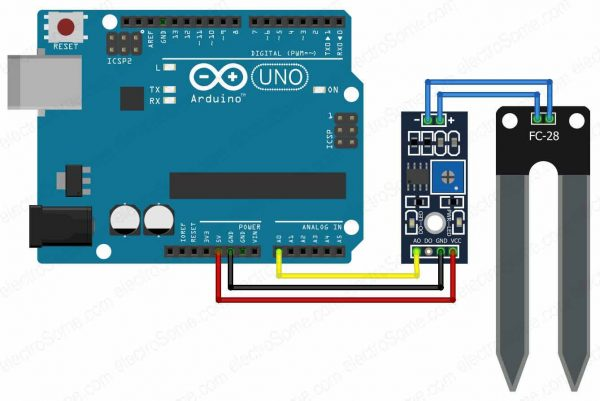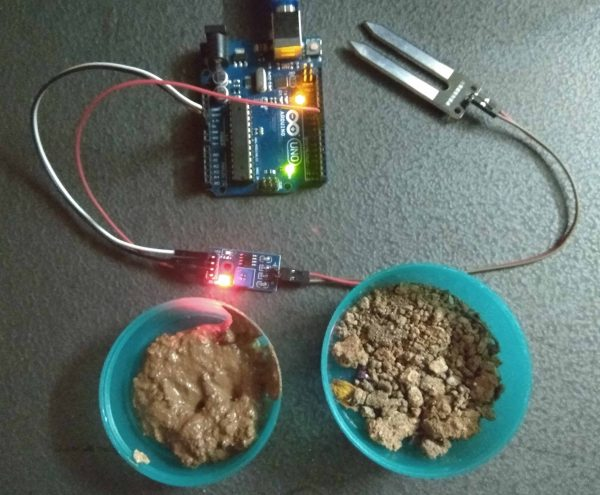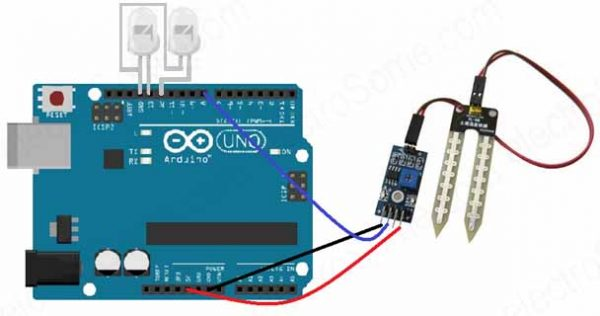# Introduction

In this article, we are going to interface a Soil Moisture Sensor with Arduino Uno. We will measure the moisture content of soil in pot or farming field and by using this we can pour water into field based on preset values.

This sensor measures the volumetric content of water inside the soil (based on resistance/conductivity) and gives the moisture level as output. The sensor is equipped with both analog and digital output, so it can be used in both analog and digital mode.

# Moisture Sensor

Soil moisture is basically the content of water present in the soil. The soil moisture sensor consists of two conducting plates which function as a probe. This sensor is used to measure the volumetric content of water.

It can measure the moisture content in the soil based on the change in resistance between these two conducting plates. It uses capacitance to measure the dielectric constant of the soil. Dielectric constant can be called as the ability of soil to transmit electricity.

When there is more water, the soil will conduct more electricity which means that there will be less resistance. Therefore, the moisture level will be higher.

Similarly dry soil conducts less electricity. When there will be less water, then the soil will conduct less electricity which means that there will be more resistance. Therefore, the moisture level will be lower.

## Specifications

The specifications of the soil moisture sensor FC-28 are as follows

Parameter Value
Input Voltage 3.3 – 5V
Output Voltage 0 – 4.2V
Input Current 35mA
Output Signal Both Analog (A0) and Digital(D0)

The Module also contains a potentiometer which will set the threshold value and then this threshold value will be compared by the LM393 comparator which is used for digital output. The output LED will light according to this threshold value.

# Operating Modes

As explained above this sensor can be connected in two modes: Analog Mode and Digital Mode. First, we will connect it in Analog mode and then we will use it in Digital mode.

## Analog Mode

### Circuit Diagram

To make it to operate in Analog Mode, we need to connect analog output of the sensor to ADC input of the Arduino. While monitoring the sensor we will get ADC values from 0-1023. The moisture content will be measured in percentage, so we have to map the values from 0 -100 and then that will be displayed on Serial Monitor.

We can further set different ranges of the moisture values and turn on or off the water pump according to it.Interfacing Soil Moisture Sensor with Arduino – Circuit Diagram

### Software

#### Arduino Code

```int sensor_pin = A0;
int output_value ;

void setup()
{
Serial.begin(9600);
delay(2000);
}

void loop()
{
output_value = map(output_value,550,0,0,100);
Serial.print("Mositure : ");
Serial.print(output_value);
Serial.println("%");
delay(1000);
}```

#### Code Explanation

Here we have defined two variables; one for the soil moisture sensor pin and the other for storing the output of the sensor.

```int sensor_pin = A0; // Soil Sensor input at Analog PIN A0
int output_value ;
```

In the setup function, the “Serial.begin(9600)” command we are initializing the serial port of Arduino to display information in the serial monitor. Then, we will print the “Reading From the Sensor …” on the serial monitor.

```void setup()
{
Serial.begin(9600);
delay(2000);
}
```

In the loop function, we will read from the sensor analog pin and will store the values in the “output_ value” variable. Then, we will map the output values to 0-100, because the moisture is measured in percentage. When we took the readings from the dry soil, then the sensor value was 550 and in the wet soil, the sensor value was 10. So, we mapped these values to get the moisture. After that, we printed these values on the serial monitor.

```void loop()
{
output_value = map(output_value,550,0,0,100);
Serial.print("Mositure : ");
Serial.print(output_value);
Serial.println("%");
delay(1000);
}```

### Practical ImplementationInterfacing Moisture Sensor with Arduino Analog Mode – Practical Implementation

## Digital Mode

To connect the soil moisture sensor FC-28 in the digital mode, we have to use digital output of the sensor and a digital input of Arduino. While monitoring the sensor gives us true or false condition with respect to measured moisture content in a soil. We can adjust the threshold using the potentiometer in the sensor.

When the sensed value is less than the threshold value, then the measured soil moisture content is low and the sensor will give us LOW.

When the sensed value is greater than the threshold value, then the measured soil moisture content is high and the sensor will give us HIGH.

### Circuit Diagram

The connections for connecting the soil moisture sensor FC-28 to the Arduino in digital mode are as follows.

• VCC of Moisture Sensor to 5V of Arduino
• GND of Moisture Sensor to GND of Arduino
• D0 of Moisture Sensor to pin 8 of Arduino
• LED1 positive to pin 13 of Arduino and negative to GND of Arduino
• LED2 positive to pin 12 of Arduino and negative to GND of ArduinoInterfacing Moisture Sensor with Arduino Digital Mode – Circuit Diagram

### Software

#### Arduino Code

```int led_pin1 =13;
int led_pin2 =12;
int sensor_pin =8;

void setup()
{
pinMode(led_pin1, OUTPUT);
pinMode(led_pin2, OUTPUT);
pinMode(sensor_pin, INPUT);
}

void loop()
{
{
digitalWrite(led_pin1, HIGH);
digitalWrite(led_pin2, LOW);
delay(2000);
}
else
{
digitalWrite(led_pin1, LOW);
digitalWrite(led_pin2, HIGH);
delay(2000);
}
}```

#### Code Explanation

First of all, we have initialized two variable for connecting the LED pin and the sensor digital pin.

```int led_pin1 =13;
int led_pin2 =12;
int sensor_pin =8;```

In the setup function, we have declared the LED pin as the output pin because; we will power the LED through that pin. Then, we declared the sensor pin as an input pin because the Arduino will take the values from the sensor through that pin.

```void setup()
{
pinMode(led_pin1, OUTPUT);
pinMode(led_pin2, OUTPUT);
pinMode(sensor_pin, INPUT);
}```

In the loop function, we have read from the sensor pin. If the output value of the sensor is higher than the threshold value, then the digital pin will be high and the led_pin1 will light up and led_pin2 will go off. If the sensor value is lower than the threshold value, then the led_pin1 will go off and led_pin2 will light up.

```void loop()
{
{
digitalWrite(led_pin1, HIGH);
digitalWrite(led_pin2, LOW);
delay(2000);
}
else
{
digitalWrite(led_pin1, LOW);
digitalWrite(led_pin2, HIGH);
delay(2000);
}
}```

### Practical ImplementationInterfacing Moisture Sensor with Arduino-Digital Mode- Practical Implementation

# Applications

• Agriculture Department
• Landscape Irrigation
• Gardening purpose